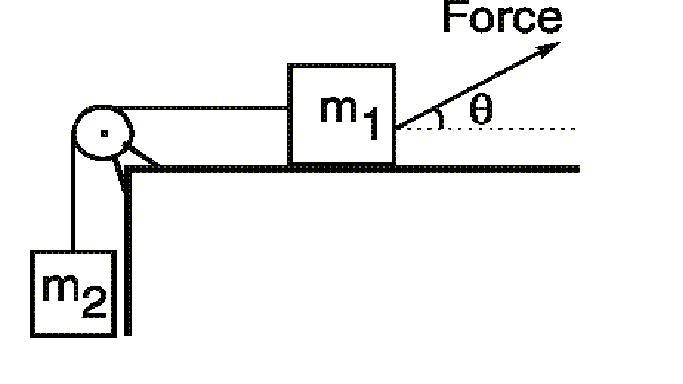# Forces and acceleration

## Homework Statement

Mass m1=31.7 kg is on a horizontal surface, connected to mass m2= 5.30 kg by a light string as shown. The pulley has negligible mass and no friction. A force of 216.9 N acts on m1 at an angle of 29.3deg. The coefficient of kinetic friction between m1 and the surface is 0.221. Determine the upward acceleration of m2.## Homework Equations

F=ma
Fn = mg-Fsin(theta)
Fcos(theta)-(uk)(Fn) = ma

## The Attempt at a Solution

First I found the normal force of M1. Fn = mg-Fsin(theta). I found Fn to be 204.513 N. Next, I found the acceleration of M1 by using [Fcos(theta)-(uk)(Fn)] / m = a. I got ax to be 4.54 m/s^2. I assumed that the acceleration would be the same for M2 but its not.

## Answers and Replies

Doc Al
Mentor
You forgot to consider the string tension acting on the block. (The accelerations of M1 and M2 must be the same--they are connected by a string.)